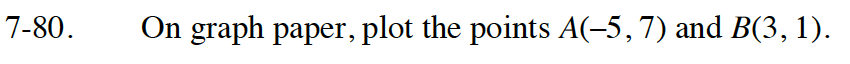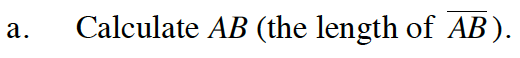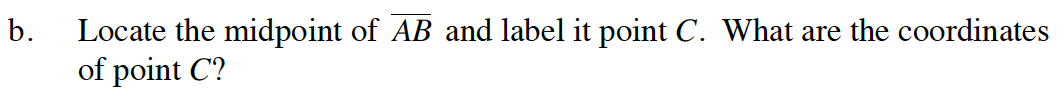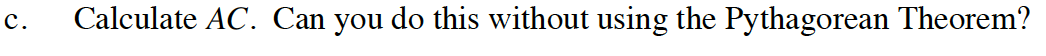Home > CC4 > Chapter 7 > Lesson 7.1.7 > Problem7-80

7-80.

On graph paper, plot the points A(–5, 7) and B(3, 1). 7-80 HW eTool (Desmos) Homework Help ✎

1. Calculate AB (the length of AB).

2. Locate the midpoint of AB and label it point C. What are the coordinates of point C?

3. Calculate AC. Can you do this without using the Pythagorean Theorem?Use the Pythagorean Theorem to find the length of AB.Use two congruent slope triangles to find the midpoint.Because C is the midpoint, what does that tell you about the length of AC?

Use the hints in the eTool below for parts (a-c).
Click on the link at right for the full eTool version: INT1 7-80 HW eTool